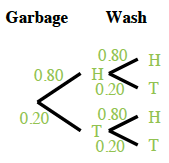### Home > INT2 > Chapter 7 > Lesson 7.1.2 > Problem7-30

7-30.

Earl hates taking out the garbage and he hates washing the dishes, so he decides to make a deal with his parents: He will flip a coin once for each chore and will perform the chore if the coin lands on heads. What he doesn’t know is that his parents are going to use a weighted coin that lands on heads $80\%$ of the time!

1. What is the probability that Earl will have to do both chores?What is the probability of flipping two heads in a row?

 Garbage Wash $\color{green}{\text{H }0.80}$ $\color{green}{\text{T }0.20}$ $\color{green}{\text{H }0.80}$ $\color{green}{0.64}$ $\color{green}{0.16}$ $\color{green}{\text{T }0.20}$

$(0.80)(0.80)=0.64=64\%$

1. What is the probability that Earl will have to take out the garbage but will not need to wash the dishes?

This is Heads for garbage and Tails for wash in your diagram.

$(0.80)(0.20)=0.16=16\%$